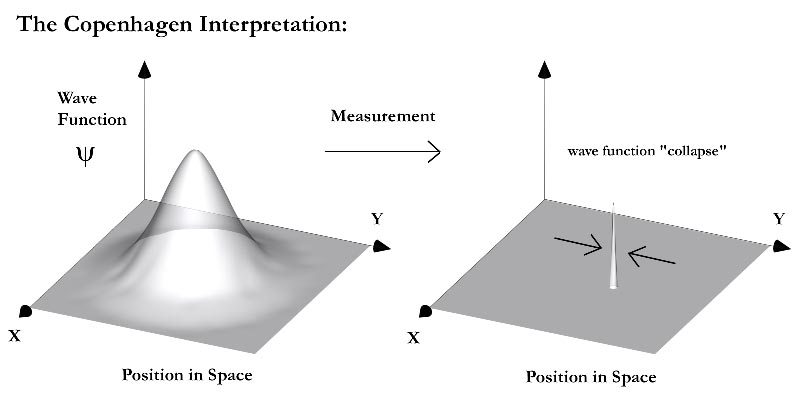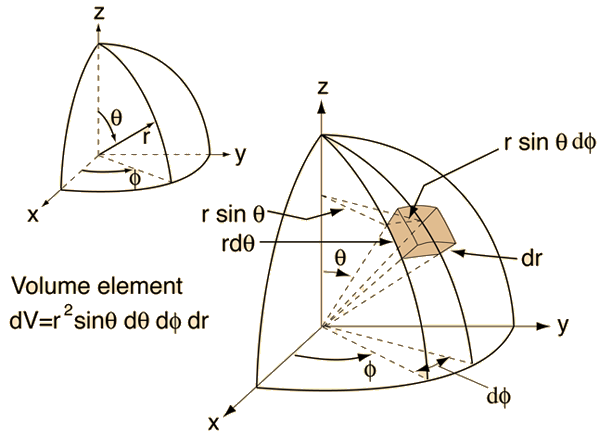# This note has been used to help create the Hydrogen Atom wikiOld rules; new tricks

The transition towards three dimensions mimics classical mechanics, where we express our equations in the $x,y,z$ coordinates . In classical mechanics, the Hamiltonian of a system is prescribed by

$H=\frac{1}{2m}\left({{p}_{x}}^{2}+{{p}_{y}}^{2}+{{p}_{z}}^{2}\right) +V(x,y,z).$

This is also true in QM, where now the time-dependent Schrödinger equation is

$i\hbar \frac{\partial \Psi}{\partial t}= -\frac{{\hbar}^{2}}{2m}{\nabla}^{2}\Psi + V\Psi$

or the time-independent case

$E\Psi= -\frac{{\hbar}^{2}}{2m}{\nabla}^{2}\Psi + V\Psi$

where ${\nabla}^{2}=\frac{{\partial}^{2}}{\partial {x}^{2}}+\frac{{\partial}^{2} }{\partial {y}^{2}}+\frac{{\partial}^{2} }{\partial {z}^{2}}$ is the Laplacian operator.

Linearity still applies in 3D! So all the linear operators used in 1D are valid:

${\hat{p}}_{x} = -i\hbar \frac{\partial}{\partial x}$

${\hat{p}}_{y} = -i\hbar \frac{\partial}{\partial y}$

${\hat{p}}_{z} = -i\hbar \frac{\partial}{\partial z}$

It is customary in physics to break a higher-dimensional problem into its directional components, solve each component and reassemble the solutions.

Since it is cumbersome to scribble down massive equations of $x,y,z$ every single time, we will switch to a more efficient notation. This notation denotes all spatial coordinates as a vector $\overrightarrow { r } =(x,y,z)$. Thus wavefunctions are now expressed as

$\Psi(\overrightarrow { r } , t) = \sum{ {c}_{n}{\psi}_{n}(\overrightarrow{r}) {e}^{-i{E}_{n}t/\hbar}}$

and potentials

$V(\overrightarrow { r }).$

As always our wavefunctions must be continuous and normalizable. In three dimensions, we integrate the probability density of the wavefunction throughout all space with volume integrals:

$\int_{V} {\Psi}^{*}{\Psi}dV =1.$

Note that many problems in physics exhibit different symmetries, in which certain coordinate systems more natural (e.g. cylindrical or spherical coordinates). Keep in mind that the geometry of a physical system dictates the appropriate methods used.Schrödinger Equation in Spherical Coordinates

Know we will briefly investigate the 3D Schrödinger equation in spherical coordinates, which is the basis for solving the hydrogen atom and atomic orbitals.

Separation of variables is a common and powerful method when confronting higher-dimensional differential equations. This is valid in whichever coordinate system $r, \theta, \phi$ we use. In spherical coordinates, consider the ansatz

$\Psi(r, \theta, \phi) = R(r)Y(\theta, \phi).$

We use the Laplacian operator in spherical coordinates: ${\nabla}^{2} = \frac{1}{{r}^{2}}\frac{\partial}{\partial r}\left({r}^{2} \frac{\partial}{\partial r}\right) + \frac{1}{{r}^{2}sin{\theta}} \frac{\partial}{\partial \theta} \left(sin{\theta} \frac{\partial}{\partial \theta}\right) + \frac{1}{{r}^{2}sin^{2}{\theta}}\left(\frac{{\partial}^{2}}{\partial {\phi}^{2}}\right)$

thus the Schrödinger equation is

$\frac{-{\hbar}^{2}}{2m}\left[\frac{1}{{r}^{2}}\frac{\partial}{\partial r}\left({r}^{2} \frac{\partial \Psi}{\partial r}\right) + \frac{1}{{r}^{2}sin{\theta}} \frac{\partial }{\partial \theta} \left(sin{\theta} \frac{\partial \Psi}{\partial \theta}\right) + \frac{1}{{r}^{2}sin^{2}{\theta}}\left(\frac{{\partial}^{2} \Psi}{\partial {\phi}^{2}}\right)\right] + V\Psi = E\Psi.$

Equivalently

$\frac{-{\hbar}^{2}}{2m}\left[\frac{Y}{{r}^{2}}\frac{\partial}{\partial r}\left({r}^{2} \frac{\partial R}{\partial r}\right) + \frac{R}{{r}^{2}sin{\theta}} \frac{\partial }{\partial \theta} \left(sin{\theta} \frac{\partial Y}{\partial \theta}\right) + \frac{R}{{r}^{2}sin^{2}{\theta}}\left(\frac{{\partial}^{2} Y}{\partial {\phi}^{2}}\right)\right] + VRY = ERY.$

Now we can clean up this equation:

$\left[\frac{1}{R}\frac{d}{dr}\left({r}^{2}\frac{dR}{dr}\right)-\frac{2m{r}^{2}}{{\hbar}^{2}}[V-E] \right]+ \frac{1}{Y}\left[\frac{1}{sin{\theta}}\frac{\partial}{\partial \theta}\left(sin{\theta}\frac{\partial Y}{\partial \theta}\right) + \frac{1}{sin^2{\theta}} \frac{{\partial}^{2}{Y}}{\partial{\phi}^{2}}\right] = 0$

Now we can separate the above equation as follows:

Radial equation $\frac{1}{R}\frac{d}{dr}\left({r}^{2}\frac{dR}{dr}\right)-\frac{2m{r}^{2}}{{\hbar}^{2}}[V-E] = l(l+1)$

Angular equation $\frac{1}{Y}\left[\frac{1}{sin{\theta}}\frac{\partial}{\partial \theta}\left(sin{\theta}\frac{\partial Y}{\partial \theta}\right) + \frac{1}{sin^2{\theta}} \frac{{\partial}^{2}{Y}}{\partial{\phi}^{2}}\right] = -l(l+1).$

But how do we solve these monsters? Well, I will not write out the solutions because they are long and quite advanced. What you should know is that we can rearrange these equations and hope that existing solutions to differential equations can be found. For a good exposition of the solutions to the radial and angular wavefunctions, and its applications to the hydrogen atom, Click here.

What is important to know is that the solutions of the angular equation contributes to the shape of atomic orbitals (angular probability density), and the solutions of the radial equation gives the radial probability density.The Hydrogen Spectrum

One of the greatest triumphs of quantum theory is the mathematical confirmation of the Hydrogen spectra. The Hydrogen spectra phenomena can be explained by electrons gaining or losing energy. In doing so, the electron changes from one stationary state to another stationary state. Usually when an electron loses energy, it gives off a photon and moves down an energy state. This difference in energy is not only observable, but quantifiable by the equation

${E}_{\gamma} = {E}_{i} - {E}_{f} = -13.6 \left( \frac{1}{{n}_{i}^{2}}-\frac{1}{{n}_{f}^{2}}\right) eV.$

But where did this equation come from? Historically, the original equation looks like this

$\frac{1}{\lambda} = R \left( \frac{1}{{n}_{f}^{2}}-\frac{1}{{n}_{i}^{2}}\right)$

which we now know can be rearranged with a bit of algebra using the Planck relation ${E}_{\gamma} = h \nu$.

This is the famous Rydberg formula, which was found empirically based on findings by Balmer (1885), Lyman (1906), and Paschen (1908). In the early 20th century, Niels Bohr formulated his theory of the Hydrogen atom and discovered that the Rydberg constant can in fact be calculated with fundamental constants

$R = \frac{{m}_{e}{e}^{4}}{8{\epsilon}_{0}^{2}{h}^{3}c} = 1.097 \times {10}^{7} {m}^{-1}.$

Visit my set Lectures on Quantum Mechanics for more notes.

ProblemsNote by Steven Zheng
6 years, 3 months ago

This discussion board is a place to discuss our Daily Challenges and the math and science related to those challenges. Explanations are more than just a solution — they should explain the steps and thinking strategies that you used to obtain the solution. Comments should further the discussion of math and science.

When posting on Brilliant:

• Use the emojis to react to an explanation, whether you're congratulating a job well done , or just really confused .
• Ask specific questions about the challenge or the steps in somebody's explanation. Well-posed questions can add a lot to the discussion, but posting "I don't understand!" doesn't help anyone.
• Try to contribute something new to the discussion, whether it is an extension, generalization or other idea related to the challenge.
• Stay on topic — we're all here to learn more about math and science, not to hear about your favorite get-rich-quick scheme or current world events.

MarkdownAppears as
*italics* or _italics_ italics
**bold** or __bold__ bold
- bulleted- list
• bulleted
• list
1. numbered2. list
1. numbered
2. list
Note: you must add a full line of space before and after lists for them to show up correctly
paragraph 1paragraph 2

paragraph 1

paragraph 2

[example link](https://brilliant.org)example link
> This is a quote
This is a quote
    # I indented these lines
# 4 spaces, and now they show
# up as a code block.

print "hello world"
# I indented these lines
# 4 spaces, and now they show
# up as a code block.

print "hello world"
MathAppears as
Remember to wrap math in $$ ... $$ or $ ... $ to ensure proper formatting.
2 \times 3 $2 \times 3$
2^{34} $2^{34}$
a_{i-1} $a_{i-1}$
\frac{2}{3} $\frac{2}{3}$
\sqrt{2} $\sqrt{2}$
\sum_{i=1}^3 $\sum_{i=1}^3$
\sin \theta $\sin \theta$
\boxed{123} $\boxed{123}$

## Comments

There are no comments in this discussion.

×

Problem Loading...

Note Loading...

Set Loading...